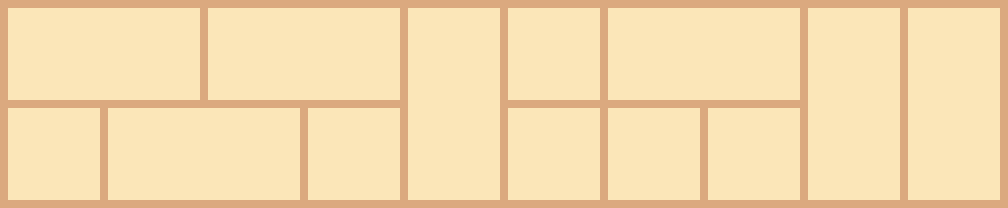# Tiling Problem

How many ways can a $2 \times 10$ rectangle be filled with $1 \times 1$ and $2 \times 1$ tiles?

One such possible tiling is shown below.Note: Rotations are allowed, so the $2 \times 1$ tiles can be placed either horizontally or vertically. This problem is intended to be solved with programming.

×